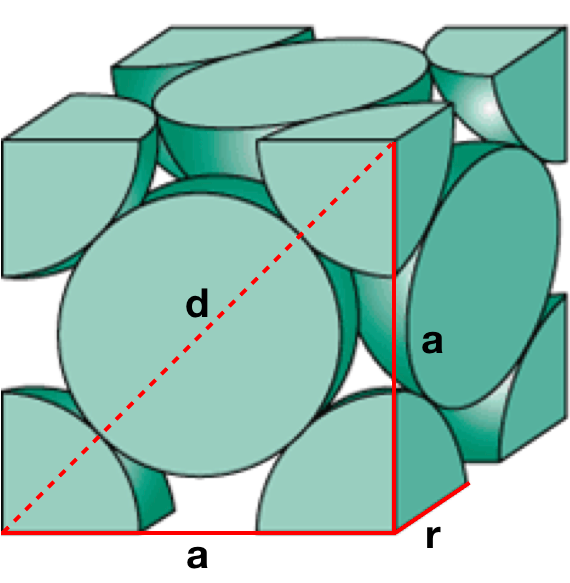# Problem: Platinum (Pt) forms a face centered cubic unit cell and has a 139 pm atomic radius. Calculate the density of platinum based on the atomic radius and unit cell.

###### FREE Expert Solution

We’re being asked to calculate the density of barium that crystallizes in a face-centered cubic unit cell.

Recall that the face-centered cubic lattice contains an atom in each of the face of the cube and an atom in each of the corners. It looks like this:Recall that density has a formula of:###### Problem Details

Platinum (Pt) forms a face centered cubic unit cell and has a 139 pm atomic radius. Calculate the density of platinum based on the atomic radius and unit cell.